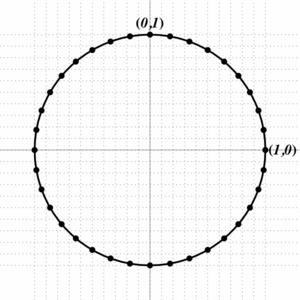# What exactly is a radian?

Alignments to Content Standards: F-TF.A.1

Definition: An angle of $1$ radian is defined to be the angle, in the counterclockwise direction, at the center of a unit circle which spans an arc of length $1.$ The picture below illustrates this definition.1. On the unit circle below, the angles $10^{\circ},\ 20^{\circ},\ 30^{\circ},$ etc., are indicated by black dots on the circle. Mark off angles of measure 0, 1, 2, 3, 4, 5, and 6 radians. Estimate the corresponding angle measure in degrees.2. Estimate the angles in radians that correspond to $180^{\circ}$ and $360^{\circ}.$

## IM Commentary

Radians are often mysterious to students, yet they are a very straight forward way to measure an angle by relating the measure of the angle to the length of the arc on the unit circle it subtends. With this definition, the angles that are easily described in radians have units of $\pi$ - a full revolution of the circle has an angle of $2\pi$ radians. This task is not designed to discover the definition of radian, rather it allows students to make meaning out of the definition. The idea is that students use a piece of string to measure the radius of the circle and then mark off the corresponding arc length on the unit circle as many times as they need to for 0, 1, 2, 3, 4, 5, and 6 radians. They then can use the black dots to estimate the corresponding angle measure in degrees.

The task does not specifically mention the use of string. Students can use a ruler or a piece of paper to "bend the radius around the circle". This gives an opportunity to use appropriate tools and talk about precision (SMPÂ 5 and 6).Â

A different solution method takes advantage of transformations, specifically rotations. In this solution we cut out the picture of a unit circle with one radian marked and put it on top of the unit circle on the grid. Once one radian is marked off on the circle, we can mark off 2, 3, 4,... radians (approximately) by rotating the cut out circle so that the old starting point of the 1 radian arc sits on top of the old end point.Â

After students find the approximate radian measure for $180^{\circ}$ and $360^{\circ}$ the teacher can point out that the exact corresponding angles in radians are $\pi$ and $2\pi,$ respectively.

## Solution

1. We can use a piece of string or a bendable ruler to measure the radius of the circle and trace the same distance around the circumference of the circle for 1, 2, 3, 4, 5, and 6 radians. The diagram below shows the corresponding angles.2. We can see from the diagram that $180^{\circ}$ corresponds to a little bit more than 3 radians. In fact, we can use the 10 degree points to help us get a better estimate. The arc subtended by 1 radian is divided into a little less than 6 equal pieces by the points and the arc subtended by 3 radians is about 1 piece short of half the circle, so $180^{\circ}$ is about $3+\frac{1}{6} \approx 3.17$ radians. Similarly, $360^{\circ}$ is about 6.34 radians: these are both slight over estimates since the arc subtended by 3 radians is a little bit less than 1 piece short of $180^\circ$.